## Ratio and Proportion For SBI PO : Set – 05

1) salaries of A, B, C are in the ratio 5:3:2. If the salaries are increased by 20%, 33 1/3% and 100% respectively. What will be the new ratios of their salaries?

a) 5:3:2

b) 2:3:2

C) 3:2:2

d) 2:2:3

e) 2:2:2

c)

Initial ratio =5 : 3 : 2

A = 5x; B=3x; C=2x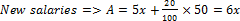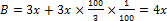C= 2x + 2x =4x

New ration =6x : 4x : 4x => 3 : 2: 2

2) The ratio between the length and width of the rectangular field is 3 : 2. If only length is increased by 5 m. The new area of the field is 2600 m^2.What is the width of the rectangular field?

a) 60 m

b) 50 m

c) 40 m

d) 65 m

e) 45m

c)

Length = 3x

Width = 2x

So (3x + 5) 2x = 2600

(3x + 5) x = 1300

3x2 + 5x -1300= 0

on solving, we get x= 20

Width 2x = 40m

3) If the ratio of areas of two squares is 225:256, then the ratio of their perimeters is:

a) 256:225

b) 225:256

c) 16:15

d) 15:16

e) 225:16

d)

Explanation

(d)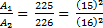Perimeter1/perimeter = (15/16)

4) An office opens at 10 AM and closes at 5 PM. The lunch interval is 30 minutes. The ratio of lunch interval to the total period of office hours is

a) 1:7

b) 1:14

c) 7:1

d) 14:1

e) None of these

b)

Working hours = 10AM to 5PM = totally 7hrs.

Lunch interval = 30 mins.

Ratio of lunch interval to total period of office hours = 7hrs: 1/2hrs  = 14:1

5) A sum of money is to be divided among 3 friends A, B C in the ratio 3:4:5. If C receives Rs. 300 as his share. What is the total amount?

a) 180

b) 240

c) 300

d) 720

e) 810

d)

Ratio of profits = 3:4:5

3 : 4 : 5

Value of C = 5 = 300

= 60

Total profit = 12 = 12 * 60 = 720

6) The respective ratio between the present age of Aarti and savita is 5:x. Aarti is 9 years younger than jahnavi. Jahnavi’s age after 9 years will be 33 years.The difference between Savita’s and Aarti’s age is same as the present age of Jahnavi.what will come in plac of x?

a) 21 years

b) 37 years

c) 42 years

d) 17 years

e) None of these

e)

Given;

Aarthi: Savitha = 5: X

Aarthi = J – 9

Jahnavi present age = 33 – 9 = 24 years

Aarthi = 24 – 9 = 15 years

Savitha – Aarthi = 24

Savitha = 24 + 15

Savitha = 39 years

Hence x = 13

7) In what ratio should a 12% methyl alcohol solution be mixed with 30% methyl alcohol solution so that the resultant solution has 20% methyl alcohol in it?

a) 1:2

b) 4:3

c) 5:4

d) 3:4

e) None of these

C)

0.12x+0.3(1-x)=0.2

0.12x+0.3-0.3x=0.2

0.1=0.8x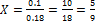1-x=1-5/9=4/9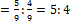8) A right circular cone is separated into solids of volumes by two planes cutting the height in the ratio of 2:1:1 from the top. Then what will be the ratio of the volumes of the frustums formed.

a) 19:37

b) 27:37

c) 8:19

d) 37:64

e) None of these

a)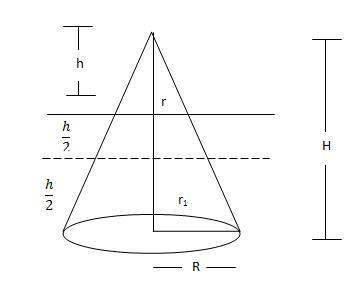{h/r=r/h(General Condition)}

1st case

h/H=r/R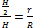r=R/2

2nd case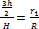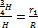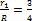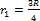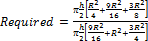= 19/37

9) The population of a state in the year 2013 was 1,12,926. If the rate of increase was 10% and 20% respectively from the year 2011 to 2012 and 2012 to 2013, what was the population in the year 2011?

a) 85,550

b) 86,450

c) 79,550

d) 75,550

e) 76,950

(a)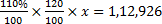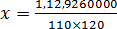x = 85550

10) The respective ratio between the present age of Aarti and Savita is 5 : . Aarti is 9 years younger than Jahnavi. Jahnavi’s age after 9 years will be 33 years.The difference between Savita’s and Aarti’s age is same as the present age of Jahnavi. What will come in place of ?

a) 21

b) 37

c) 17

d) cannot be determined

e) None of these

e)

Jahnavi’s present age =33 – 9o

=24 y

Aarti’s present age = 24 – 9

=15 yr

Aarti : Savita = 5 : x =15 : 3x

Savita’s present age = 3x yr

3x – 15 =24

3x =24 + 15 =39

x = 13